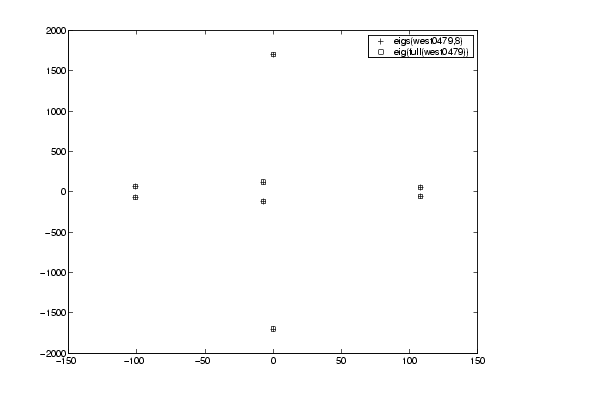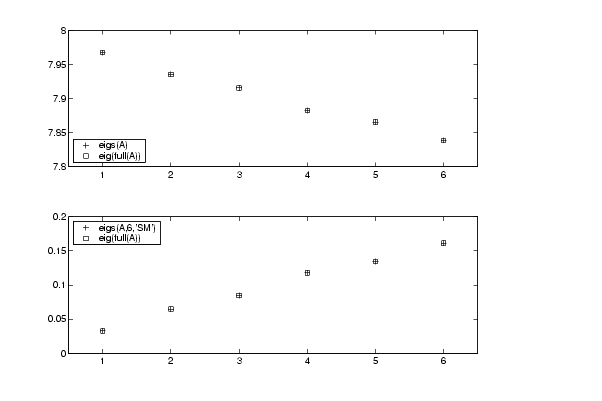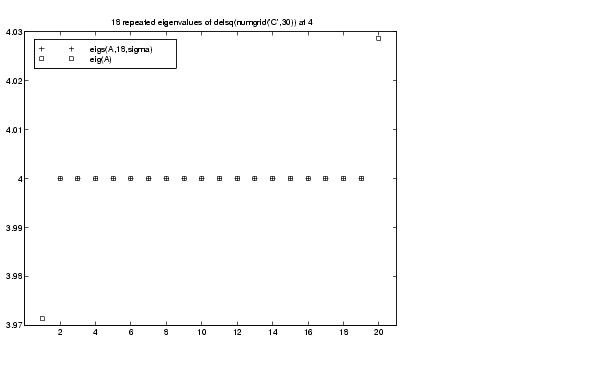MATLAB Function Referenceeigs

Find largest eigenvalues and eigenvectors of a sparse matrix

Syntax

• ```d = eigs(A)
d = eigs(A,B)
d = eigs(A,k)
d = eigs(A,B,k)
d = eigs(A,k,sigma)
d = eigs(A,B,k,sigma)
d = eigs(A,k,sigma,options)
d = eigs(A,B,k,sigma,options)
d = eigs(Afun,n)
d = eigs(Afun,n,B)
d = eigs(Afun,n,k)
d = eigs(Afun,n,B,k)
d = eigs(Afun,n,k,sigma)
d = eigs(Afun,n,B,k,sigma)
d = eigs(Afun,n,k,sigma,options)
d = eigs(Afun,n,B,k,sigma,options)
[V,D] = eigs(A,...)
[V,D] = eigs(Afun,n,...)
[V,D,flag] = eigs(A,...)
[V,D,flag] = eigs(Afun,n,...)
```

Description

```d = eigs(A) ``` returns a vector of `A`'s six largest magnitude eigenvalues.

```[V,D] = eigs(A) ``` returns a diagonal matrix `D` of `A`'s six largest magnitude eigenvalues and a matrix `V` whose columns are the corresponding eigenvectors.

```[V,D,flag] = eigs(A) ``` also returns a convergence flag. If `flag` is `0` then all the eigenvalues converged; otherwise not all converged.

```eigs(A,B) ``` solves the generalized eigenvalue problem `A*V == B*V*D`. `B` must be symmetric (or Hermitian) positive definite and the same size as `A`. `eigs(A,[],...)` indicates the standard eigenvalue problem `A*V == V*D`.

```eigs(A,k) and eigs(A,B,k) ``` return the `k` largest magnitude eigenvalues.

```eigs(A,k,sigma) and eigs(A,B,k,sigma) ``` return `k` eigenvalues based on `sigma`, which can take any of the following values:

 scalar (real or complex, including 0) The eigenvalues closest to `sigma`. If `A` is a function, `Afun` must return `Y = (A-``sigma``*B)\x` (i.e., `Y = A\x` when `sigma`` = 0`). Note, `B` need only be symmetric (Hermitian) positive semi-definite. `'lm'` Largest magnitude (default). `'sm'` Smallest magnitude. Same as `sigma`` = 0`. If `A` is a function, `Afun` must return `Y = A\x`. Note, `B` need only be symmetric (Hermitian) positive semi-definite. For real symmetric problems, the following are also options: `'la'` Largest algebraic (`'lr'` in MATLAB 5) `'sa'` Smallest algebraic (`'sr'` in MATLAB 5) `'be'` Both ends (one more from high end if `k` is odd) For nonsymmetric and complex problems, the following are also options: `'lr'` Largest real part `'sr'` Smallest real part `'li'` Largest imaginary part `'si'` Smallest imaginary part

 Note    The MATLAB 5 value `sigma`` = 'be'` is obsolete for nonsymmetric and complex problems.

 Note    The syntax `eigs(A,k,...)` is not valid when `A` is scalar. To pass a value for `k`, you must specify `B` as the second argument and `k` as the third (`eigs(A,B,k,...)`). If necessary, you can set `B` equal to `[]`, the default.

```eigs(A,K,sigma,opts) and eigs(A,B,k,sigma,opts) ``` specify an options structure. Default values are shown in brackets (`{}`).

 Parameter Description Values `options.issym` `1` if `A` or `A-``sigma``*B` represented by `Afun` is symmetric, `0` otherwise. `[{0} | 1]` `options.isreal` `1` if `A` or `A-``sigma``*B` represented by `Afun` is real, `0` otherwise. `[0 | {1}]` `options.tol` Convergence: Ritz estimate residual `<= tol*norm(A)`. `[scalar | {eps}]` `options.maxit` Maximum number of iterations. `[integer | {300}]` `options.p` Number of basis vectors. `p >= 2k` (`p >= 2k+1` real nonsymmetric) advised. Note: `p` must satisfy `k < p <= n` for real symmetric, `k+1 < p <= n` otherwise. `[integer | 2*k]` `options.v0` Starting vector. `Randomly generated by ARPACK` `options.disp` Diagnostic information display level. `[0 | {1} | 2]` `options.cholB` `1` if `B` is really its Cholesky factor `chol(B)`, `0` otherwise. `[{0} | 1]` `options.permB` Permutation vector `permB` if sparse `B` is really `chol(B(permB,permB))`. `[permB | {1:n}]`

 Note    MATLAB 5 options `stagtol` and `cheb` are no longer allowed.

```eigs(Afun,n,...) ``` accepts the function handle `Afun` instead of the matrix `A`. See Function Handles in the MATLAB Programming documentation for more information. `Afun` must accept an input vector of size `n`.

`y = Afun(x)` should return:

 `A*x` if `sigma` is not specified, or is a string other than `'sm'` `A\x` if `sigma` is `0` or `'sm'` `(A-sigma*I)\x` if `sigma` is a nonzero scalar (standard eigenvalue problem). `I` is an identity matrix of the same size as `A`. `(A-sigma*B)\x` if `sigma` is a nonzero scalar (generalized eigenvalue problem)

Parameterizing Functions Called by Function Functions, in the MATLAB mathematics documentation, explains how to provide additional parameters to the function `Afun`, if necessary.

The matrix `A`, `A-``sigma``*I` or `A-``sigma``*B` represented by `Afun` is assumed to be real and nonsymmetric unless specified otherwise by `opts.isreal` and `opts.issym`. In all the `eigs` syntaxes, `eigs(A,...)` can be replaced by `eigs(Afun,n,...)`.

Remarks

`d = eigs(A,k)` is not a substitute for

• ```d = eig(full(A))
d = sort(d)
d = d(end-k+1:end)
```

but is most appropriate for large sparse matrices. If the problem fits into memory, it may be quicker to use `eig(full(A))`.

Algorithm

`eigs` provides the reverse communication required by the Fortran library ARPACK, namely the routines `DSAUPD`, `DSEUPD`, `DNAUPD`, `DNEUPD`, `ZNAUPD`, and `ZNEUPD`.

Examples

Example 1.

• ```A = delsq(numgrid('C',15));
d1 = eigs(A,5,'sm')
```

returns

• ```Iteration 1: a few Ritz values of the 20-by-20 matrix:
0
0
0
0
0

Iteration 2: a few Ritz values of the 20-by-20 matrix:
1.8117
2.0889
2.8827
3.7374
7.4954

Iteration 3: a few Ritz values of the 20-by-20 matrix:
1.8117
2.0889
2.8827
3.7374
7.4954

d1 =

0.5520
0.4787
0.3469
0.2676
0.1334
```

Example 2. This example replaces the matrix `A` in example 1 with a handle to a function `dnRk`. The example is contained in an M-file `run_eigs` that

• Calls `eigs` with the function handle `@dnRk` as its first argument.
• Contains `dnRk` as a nested function, so that all variables in `run_eigs` are available to `dnRk`.

The following shows the code for `run_eigs`:

• ```function d2 = run_eigs
n = 139;
opts.issym = 1;
R = 'C';
k = 15;
d2 = eigs(@dnRk,n,5,'sm',opts);

function y = dnRk(x)
y = (delsq(numgrid(R,k))) \ x;
end
end
```

Example 3. `west0479` is a real 479-by-479 sparse matrix with both real and pairs of complex conjugate eigenvalues. `eig` computes all 479 eigenvalues. `eigs` easily picks out the largest magnitude eigenvalues.

This plot shows the 8 largest magnitude eigenvalues of `west0479` as computed by `eig` and `eigs`.

• ```load west0479
d = eig(full(west0479))
dlm = eigs(west0479,8)
[dum,ind] = sort(abs(d));
plot(dlm,'k+')
hold on
plot(d(ind(end-7:end)),'ks')
hold off
legend('eigs(west0479,8)','eig(full(west0479))')```

Example 4. `A = delsq(numgrid('C',30))` is a symmetric positive definite matrix of size 632 with eigenvalues reasonably well-distributed in the interval (0 8), but with 18 eigenvalues repeated at 4. The `eig` function computes all 632 eigenvalues. It computes and plots the six largest and smallest magnitude eigenvalues of `A` successfully with:

• ```A = delsq(numgrid('C',30));
d = eig(full(A));
[dum,ind] = sort(abs(d));
dlm = eigs(A);
dsm = eigs(A,6,'sm');

subplot(2,1,1)
plot(dlm,'k+')
hold on
plot(d(ind(end:-1:end-5)),'ks')
hold off
legend('eigs(A)','eig(full(A))',3)
set(gca,'XLim',[0.5 6.5])

subplot(2,1,2)
plot(dsm,'k+')
hold on
plot(d(ind(1:6)),'ks')
hold off
legend('eigs(A,6,''sm'')','eig(full(A))',2)
set(gca,'XLim',[0.5 6.5])```

However, the repeated eigenvalue at 4 must be handled more carefully. The call `eigs(A,18,4.0)` to compute 18 eigenvalues near 4.0 tries to find eigenvalues of `A - 4.0*I`. This involves divisions of the form `1/(lambda - 4.0)`, where `lambda` is an estimate of an eigenvalue of `A`. As `lambda` gets closer to 4.0, `eigs `fails. We must use `sigma` near but not equal to 4 to find those 18 eigenvalues.

• ```sigma = 4 - 1e-6
[V,D] = eigs(A,18,sigma)
```

The plot shows the 20 eigenvalues closest to 4 that were computed by `eig`, along with the 18 eigenvalues closest to `4 - 1e-6` that were computed by `eigs`.See Also

`arpackc`, `eig`, `svds`, `function_handle` (`@`)

References

  Lehoucq, R.B. and D.C. Sorensen, "Deflation Techniques for an Implicitly Re-Started Arnoldi Iteration," SIAM J. Matrix Analysis and Applications, Vol. 17, 1996, pp. 789-821.

  Lehoucq, R.B., D.C. Sorensen, and C. Yang, ARPACK Users' Guide: Solution of Large-Scale Eigenvalue Problems with Implicitly Restarted Arnoldi Methods, SIAM Publications, Philadelphia, 1998.

  Sorensen, D.C., "Implicit Application of Polynomial Filters in a k-Step Arnoldi Method," SIAM J. Matrix Analysis and Applications, Vol. 13, 1992, pp. 357-385.

© 1994-2005 The MathWorks, Inc.